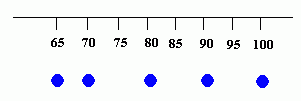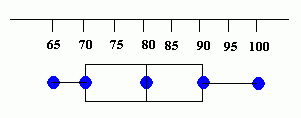Name: ___________________Date:___________________

 Email us to get an instant 20% discount on highly effective K-12 Math & English kwizNET Programs!

High School Mathematics - 211.4 Box and Whisker Plots

 Data can be displayed in many ways. One method of displaying a set of data is with a box-and-whisker plot. Box-and-whisker plots: are helpful in interpreting the distribution of data. are used to summarize data and to illustrate the variability of the data. is a pictorial representation of the variability of the data. The plots graphically display the median, quartiles, inter-quartile range and extreme values in a set of data. They can be drawn vertically or horizontally. It consists of rectangular box with the ends or hinges located at the first and third quartiles. The segments extending from the ends of the box are called whiskers. The whiskers stop at the extreme values of the set, unless the set contains outliners. Outliners are extreme values that are more than 1.5 times the interquartile range beyond the upper and lower quartiles. Outliners are represented by single point. If an outliner exists, each whisker is extended to the last value of the data that is not an outliner. The dimensions of the box-and-whisker plot help characterize the data. Each whisker and each small box contains 25% of the data. If the whisker or box is short, the data are concentrated over a narrower range of values. The longer the whisker or box, the larger the range of the data in that quartile. If the data is arranged in order and the median is found, the set of data is divided into two groups. If the median of each group is found, the data is divided into two groups. Each of these groups is called a quartile. Quartiles separate the original set of data into four equal parts. Each of these parts contains one-fourth of the data. There are three quartile points Q1, Q2, Q3 that denote the breaks in the data for each quartile. The median is the second quartile point Q2. The medians of the two groups defined by the median are the first quartile points Q1 and the third Q3. One fourth of the data is less than the first quartile point Q1, and three fourth of the data is less than the third quartile point Q3. The difference between the first quartile point and third quartile point is called the interquartile range. When the interquartile range is dived by 2, the quotient is called the semi-interquartile range. Example: Math test scores of Mrs B's class are given as: 80, 75, 90, 95, 65, 65, 80, 85, 70, 100. Constructing a box-and-whisker plot for the data. Write the data in ascending order and find the first quartile, the median, the third quartile, the smallest value and the largest value. 65, 65, 70, 75,80, 80, 85, 90, 95, 100 median Q2 = 80 first quartile Q1 = 70 third quartile Q3= 90 smallest value = 65 largest value = 100 Place a circle beneath each of these values on a number line.Draw a box with ends through the points for the first and third quartiles. Then draw a vertical line through the box at the median point. Then, draw the whiskers (or lines) from each end of the box to the smallest and largest values.Directions: Answer the following questions. Also draw a box-and-whisker plot for the following data set: 4.3, 5.1, 3.9, 4.5, 4.4, 4.9, 5.0, 4.7, 4.1, 4.6, 4.4, 4.3, 4.8, 4.4, 4.2, 4.5, 4.4 77, 79, 80, 86, 87, 87, 94, 99 79, 53, 82, 91, 87, 98, 80, 93Name: ___________________Date:___________________

High School Mathematics - 211.4 Box and Whisker Plots

 Q 1: Find the interquartile range of the college enrollments: 17,090 18,001 19,886 20,325 20925 21,498 21,645 21,970 22,393 22,836 24,075 24,141, 24,481 27,874 28,285 29,693 37,718 45,462 47,476Answer: Q 2: The difference between the first quartile point and third quartile point is called __________ range.Answer: Q 3: When the interquartile range is divided by 2, the quotient is called the _____-interquartile range.Answer: Q 4: If a set of data has first quartile point Q1 and third quartile point Q3, the semi-interquartile range QR is ________.(Q2- Q1)/3(Q3+ Q1)/2(Q1- Q2)/2(Q3- Q1)/2 Q 5: Find the median of the following data: 77, 79, 80, 86, 87, 87, 94, 99 Answer: Q 6: Find the median of the following data: 4.3, 5.1, 3.9, 4.5, 4.4, 4.9, 5.0, 4.7, 4.1, 4.6, 4.4, 4.3, 4.8, 4.4, 4.2, 4.5, 4.4Answer: Q 7: The ________ quartile is another name for the median of the entire set of data. Answer: Q 8: The measures of variability is the __________.Answer: Question 9: This question is available to subscribers only! Question 10: This question is available to subscribers only!

Subscription to kwizNET Learning System costs less than \$1 per month & offers the following benefits:

• Unrestricted access to grade appropriate lessons, quizzes, & printable worksheets
• Instant scoring of online quizzes
• Progress tracking and award certificates to keep your student motivated
• Unlimited practice with auto-generated 'WIZ MATH' quizzes